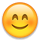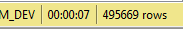Hello Masters .. I'm using datatables (v.1.10.23) and CodeIgniter(v.3) in my project and everything is cool .. and one of my module has a large data (480,879 record). only in that page ajax get pending when i open it and when the pending is disappeared it takes around 14-15sec for loading the data, other pages with it takes less than 1sec or 2sec.

and I set only 5 data per page .. I was though that can make it faster little bit but nothing changed..

so I hope someone can help me to solve it . I don't know if there something wrong in my code

Here is my ajax datatbales :
`function datatable(){`
`\$('#Table3').DataTable({`
`"processing": true,`
`"serverSide": true,`
`"pageLength":5,`
`"deferRender": true,`
`"responsive":true,`
`"order": [[ 0, "desc" ]],`
`"ajax":{"url": "<?php echo base_url().'Penjualan/get_ajax';?>",`
`"type": "POST"`
`},`
`"createdRow": function( row, data, dataIndex ){`
`if(data != "0"){`
`\$(row).addClass('red');`
`}`
`},`
`dom:`
`"<'row'<'col-sm-12'f>>" +`
`"<'row'<'col-sm-12'tr>>" +`
`"<'row'<'col-sm-12'p>>",`
`"columnDefs": [{`
`"targets": [ 9 ],`
`"visible": false,`
`"searchable": false`
`}],`
`});`
`}`
`\$(document).ready(function(){`
`datatable();`
`});`

Here is my Controller`function get_ajax() {`
`\$list = \$this->Penjualan_model->get_datatables();`
`\$data = array();`
`\$no = @\$_POST['start'];`
`foreach (\$list as \$item) {`
`\$no++;`
`\$row = array();`
`\$row[] = \$no;`
`\$row[] = \$item['no_bukti'];`
`\$row[] = date('d-m-Y',strtotime(\$item['tgl_jual']));`
`\$row[] = \$item['kode_ctr'];`
`\$row[] = \$item['nama_ctr'];`
`\$row[] = \$item['kode_mcm'];`
`\$row[] = \$item['id_mcm'];`
`\$row[] = \$item['nama_mcm'];`
`\$row[] = \$item['no_ext'];`
`\$row[] = \$item['flag'];`
`// add html for action`
`\$row[] = '<button type="button" class="btn btn-info btn-xs open-record" data-toggle="modal" value="'.\$item['no_bukti'].'" id="view" data-no_bukti="'.trim(\$item['no_bukti']).'" data-flag="'.trim(\$item['flag']).'">` `<i class="fa fa-eye"></i></button>`

`<button type="button" class="btn btn-danger btn-xs" value="'.\$item['no_bukti'].'" data-no_bukti="'.\$item['no_bukti'].'" data-toggle="modal" data-target="#trash" id="Bin"><i class="fa fa-trash"></i></button>';`
`\$data[] = \$row;`
`}`
`\$output = array(`
`"draw" => @\$_POST['draw'],`
`"recordsTotal" => \$this->Penjualan_model->count_all(),`
`"recordsFiltered" => \$this->Penjualan_model->count_filtered(),`
`"data" => \$data,`
`);`
`// output to json format`
`echo json_encode(\$output);`
`}`

and here is my Model:
`private function _get_datatables_query(){`
`var \$column_order = array('a.tgl_jual', 'a.kode_ctr', 'b.nama_ctr', 'a.kode_mcm', 'a.id_mcm', 'c.nama_mcm');`
`var \$column_search = array('a.no_bukti','a.tgl_jual', 'a.kode_ctr', 'b.nama_ctr', 'a.kode_mcm', 'a.id_mcm', 'c.nama_mcm');`
`var \$order = array('tgl_jual' => 'asc');`
`}`

``````private function _get_datatables_query(){
\$akses = trim(\$this->session->userdata('akses'));
\$kode_ctr = trim(\$this->session->userdata('kode_ctr'));
\$this->db->select('a.*,b.nama_ctr,c.nama_mcm');
\$this->db->from('mcmjualh a');
\$this->db->join('mcmcenter b', 'a.kode_ctr = b.kode_ctr','left');
\$this->db->join('mcmmcm c', 'a.id_mcm = c.id_mcm','left');
\$this->db->where('1=1');
\$this->db->where('a.kode_ctr', \$kode_ctr);
}
\$i = 0;
foreach (\$this->column_search as \$item){
if(@\$_POST['search']['value']){
if(\$i===0){
\$this->db->group_start();
\$this->db->like(\$item, \$_POST['search']['value']);
}else{
\$this->db->or_like(\$item, \$_POST['search']['value']);
}
if(count(\$this->column_search) - 1 == \$i)
\$this->db->group_end();
}
\$i++;
}
if(isset(\$_POST['order'])){
\$this->db->order_by(\$this->column_order[\$_POST['order']['0']['column']], \$_POST['order']['0']['dir']);
}elseif(isset(\$this->order)){
\$order = \$this->order;
\$this->db->order_by(key(\$order), \$order[key(\$order)]);
}
}
function get_datatables() {
\$this->_get_datatables_query();
if(@\$_POST['length'] != -1)
\$this->db->limit(@\$_POST['length'], @\$_POST['start']);
\$query = \$this->db->get();
return \$query->result_array();
}
function count_filtered() {
\$this->_get_datatables_query();
\$query = \$this->db->get();
return \$query->num_rows();
}
function count_all() {
\$this->db->from('mcmjualh');
return \$this->db->count_all_results();
}
``````

can someone tell me what is the problem ? and is there something wrong in my code ?
sorry if my English is not realy good. because its not my mother tongue``

• I would start by outputting the SQL that that you are building and running it against the database directly. What sort of response time does it have the for queries you are running?

Allan

• it takes around 6 - 7 second if i run the query in my SQL server management studio

here is the screenshot of response time of query :• Wow - that's a long query. Can you show it to me? Also, it would be worth adding `EXPLAIN` at the start when you run it in SMS to have it tell you why it is running so slowly. Possibly a missing index?

Allan